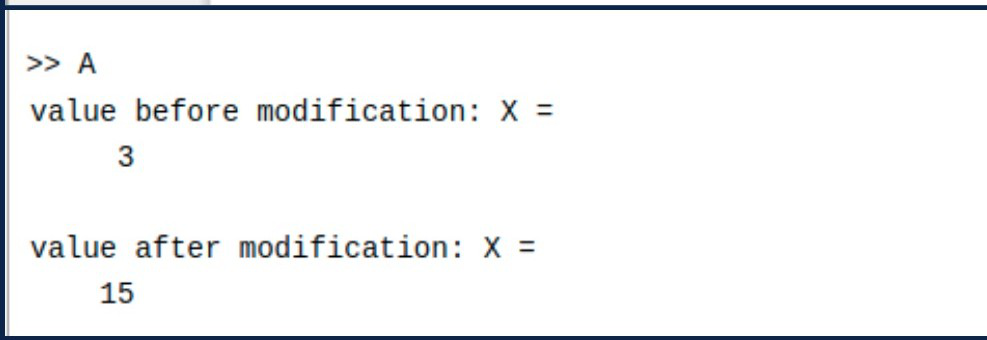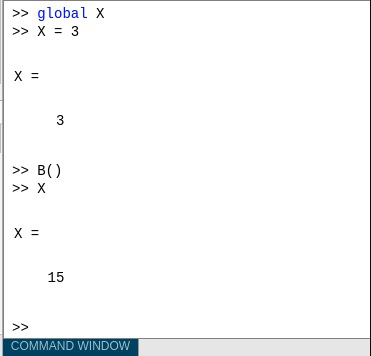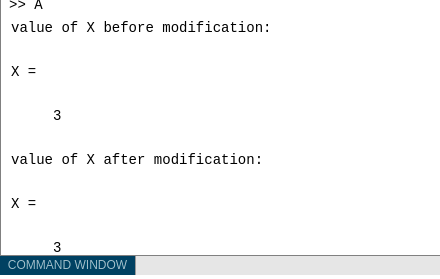Related Articles

# Global Variables in MATLAB

• Last Updated : 04 Jul, 2021

Variables in programming are generally storage spaces to store a certain type of data. There are many types of variables, but two commonly used types are local and Global variables. Generally, each MATLAB function has its own local variables. But sometimes for the sake of programming, we need to change the value of the local variable in another function. Sometimes it becomes a tedious task to perform. Here, comes the need for the global variables. If we declare any variable as global, we can access that variable from any function. Basically, all functions share the same copy of the variable. Any change of value to that variable, in any function, is visible to all the functions that declare it as global.

### Set variable as Global in MATLAB

A variable in MATLAB is set as global by writing a global command before the variable name(s). While declaring any variable as global for the first time, the variable is initialized to an empty 0X0 matrix. If any variable with the same name as a global variable is declared while the global variable exists in the current workspace MATLAB creates a warning and changes that variable and its scope to match with the global variable. We have to write the global command in every function where we want to use that global variable. Some useful tips are given below.

• If we want to clear a global variable from all workplaces, we can use the command: clear global variable.
• On the other hand, the command “clear variable” will only clear the variable from the current workspace, not from the other workspaces.

Syntax: global variable_name1 … variable_nameN

Note: Here we have declared N global variables where the name of the ith global variable is variable_namei. Generally, for the name of global variables uppercase letters are used so that, we can easily differentiate between the local and the global variables.

Suppose, we declare a global variable following the above syntax with the name global variable_name inside function A. Now, we want to modify that global variable in function B. In that case, we need to write the global command before variable_name again inside function B. Otherwise, it will not work. Following are the examples.

We take one script A.m and one function named function B is used for example.

• Share global variables between two functions

In this case, we will declare the global variable X in script A.m and will modify it inside function B and try to see the output.

Example:

## Matlab

 `% MATLAB code for function B ``% and save as MATLAB script name B.m`` ` `function` `B()``    ``global` `X``    ``% global command used ``    ``% as we want to modify the global``    ``% variable declared in A.m``    ``X = X*5;``end`

MATLAB Script A.m

## Matlab

 `% MATLAB code for main script A.m``global` `X; ``% declaring global function``X = 3;``disp(``"value of X before modification:"``);``X``B(); ``% calling B function written above.``disp(``"value of X after modification:"``);``X`

Output:• Sharing global variable between function and command line

Here we will declare the global variable in the command window and will modify it inside function B and try to see the output.

## Matlab

 `% MATLAB Code for global variable in the ``% command window and will modify it inside ``% function B for this again we use script B.M``function` `B()``global` `X``    ` `% global command used ``% as we want to modify the global``% variable declared in A.m``X = X*5;``end`

Output:• If the global command is not used in every function:

Here we will declare the global variable in script A.m and will try to modify the variable inside function B without using the command ‘global’. But in the output, we will see that the variable will not be modified as we haven’t use the command ‘global’ inside function B.

## Matlab

 `% MATLAB code for  declare the global ``% variable in script A.m and will try to``% modify the variable inside function B without``% using the command 'global'function B()`` ``function` `B()`` ``X = 3*5;``  ` ` ``end`

## Matlab

 `% MATLAB code declare the global variable in ``% script A.m and will try to modify the variable``% inside function B without using the command 'global'``global` `X; ``X = 3;`` ` `disp(``"value of X before modification:"``);``X`` ` `B(); ``% calling B function written above.``disp(``"value of X after modification:"``);``X`

Output:My Personal Notes arrow_drop_up